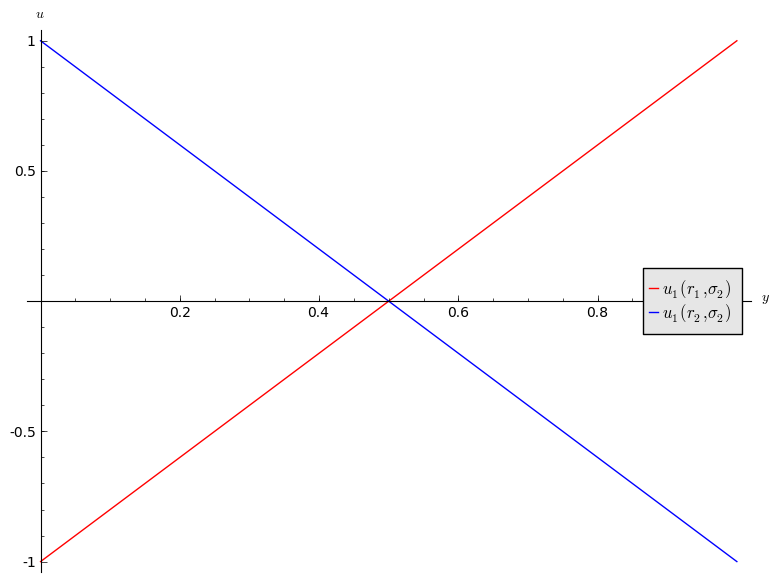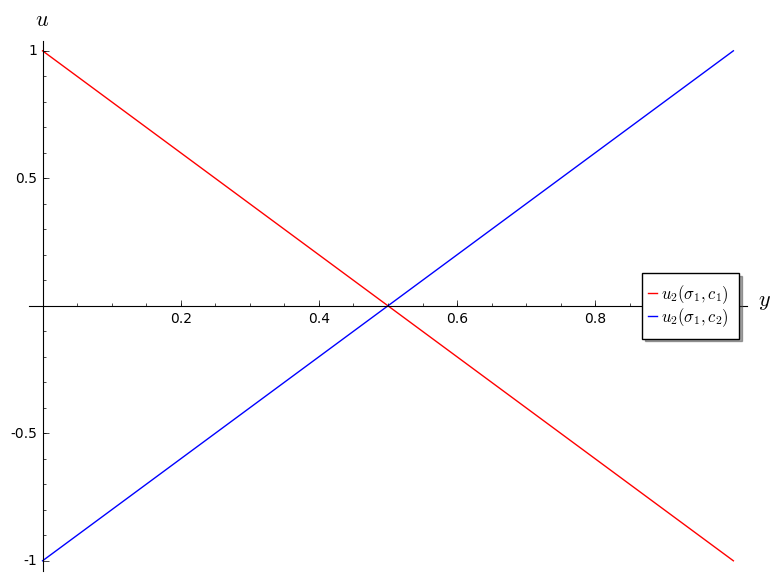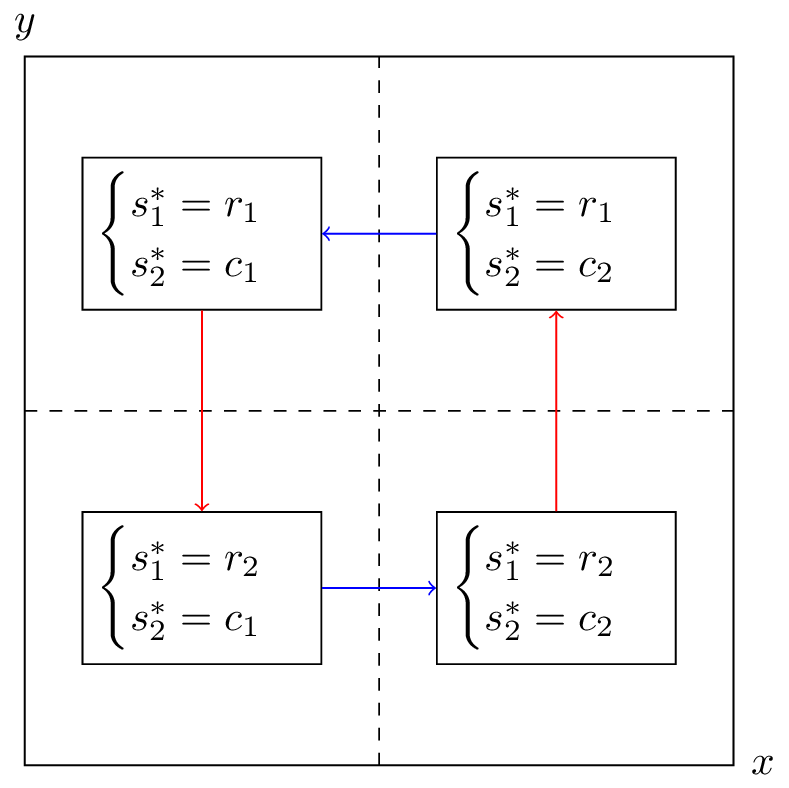## Recap

In the previous chapter

• The definition of Nash equilibria;
• Identifying Nash equilibria in pure strategies;
• Solving the duopoly game;

This brings us to a very important part of the course. We will now consider equilibria in mixed strategies.

## Recall of expected utility calculation

In the matching pennies game discussed previously:

Recalling Chapter 2 a strategy profile of $$\sigma_1=(.2,.8)$$ and $$\sigma_2=(.6,.4)$$ implies that player 1 plays heads with probability .2 and player 2 plays heads with probability .6.

We can extend the utility function which maps from the set of pure strategies to $$\mathbb{R}$$ using expected payoffs. For a two player game we have:

## Obtaining equilibria

Let us investigate the best response functions for the matching pennies game.

If we assume that player 2 plays a mixed strategy $$\sigma_2=(y,1-y)$$ we have:

$u_1(r_1,\sigma_2)=2y-1$ and $u_1(r_2,\sigma_2)=1-2y$

shown:1. If $$y<1/2$$ then $$r_2$$ is a best response for player 1.
2. If $$y>1/2$$ then $$r_1$$ is a best response for player 1.
3. If $$y=1/2$$ then player 1 is indifferent.

If we assume that player 1 plays a mixed strategy $$\sigma_1=(x,1-x)$$ we have:

$u_2(\sigma_1,c_1)=1-2x$ and $u_2(\sigma_1,c_2)=2x-1$

shown:Thus we have:

1. If $$x<1/2$$ then $$c_1$$ is a best response for player 2.
2. If $$x>1/2$$ then $$c_2$$ is a best response for player 2.
3. If $$x=1/2$$ then player 2 is indifferent.

Let us draw both best responses on a single diagram, indicating the best responses in each quadrant. The arrows show the deviation indicated by the best responses.If either player plays a mixed strategy other than $$(1/2,1/2)$$ then the other player has an incentive to modify their strategy. Thus the Nash equilibria is:

This notion of “indifference” is important and we will now prove an important theorem that will prove useful when calculating Nash Equilibria.

## Equality of payoffs theorem

### Definition of the support of a strategy

In an $$N$$ player normal form game the support of a strategy $$\sigma\in\Delta S_i$$ is defined as: $\mathcal{S}(\sigma)=\{s\in S_i\;|\;\sigma(s)>0\}$

I.e. the support of a strategy is the set of pure strategies that are played with non zero probability.

For example, if the strategy set is $${A,B,C}$$ and $$\sigma=(1/3,2/3,0)$$ then $$\mathcal{S}(\sigma)={A,B}$$.

### Theorem of equality of payoffs

In an $$N$$ player normal form game if the strategy profile $$(\sigma_i,s_{-i})$$ is a Nash equilibria then:

### Proof

If $$|\mathcal{S}(\sigma_i)|=1$$ then the proof is trivial.

We assume that $$|\mathcal{S}(\sigma_i)|>1$$. Let us assume that the theorem is not true so that there exists $$\bar s\in\mathcal{S}(\sigma)$$ such that

Without loss of generality let us assume that:

Thus we have:

Giving:

which implies that $$(\sigma_i,s_{-i})$$ is not a Nash equilibrium.

### Example

Let’s consider the matching pennies game yet again. To use the equality of payoffs theorem we identify the various supports we need to try out. As this is a $$2\times 2$$ game we can take $$\sigma_1=(x,1-x)$$ and $$\sigma_2=(y,1-y)$$ and assume that $$(\sigma_1,\sigma_2)$$ is a Nash equilibrium.

from the theorem we have that $$u_1(\sigma_1,\sigma_2)=u_1(r_1,\sigma_2)=u_1(r_2,\sigma_2)$$

Thus we have found player 2’s Nash equilibrium strategy by finding the strategy that makes player 1 indifferent. Similarly for player 1:

Thus the Nash equilibria is:

To finish this chapter we state a famous result in game theory:

### Nash’s Theorem

Every normal form game with a finite number of pure strategies for each player, has at least one Nash equilibrium.